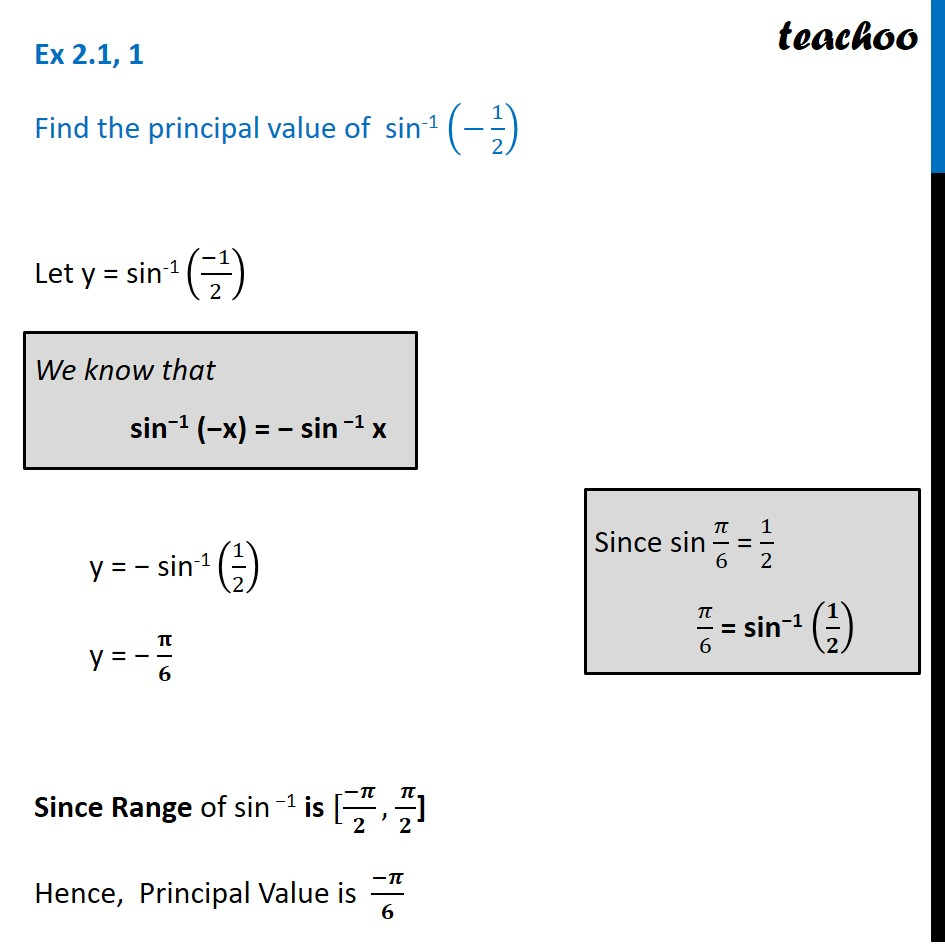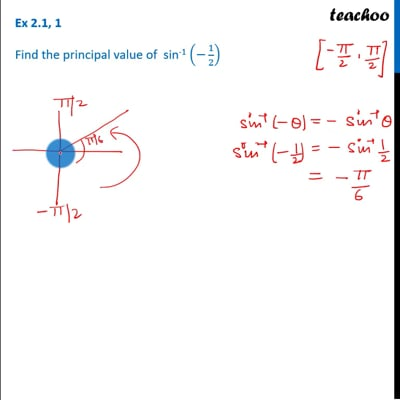Ex 2.1

Chapter 2 Class 12 Inverse Trigonometric Functions
Serial order wiseThis video is only available for Teachoo black usersThis video is only available for Teachoo black users

Learn in your speed, with individual attention - Teachoo Maths 1-on-1 Class

### Transcript

Ex 2.1, 1 Find the principal value of sin-1 (−1/2) Let y = sin-1 ((−1)/2) y = − sin-1 (1/2) y = − 𝛑/𝟔 Since Range of sin −1 is [(−𝝅)/𝟐, ( 𝝅)/𝟐] Hence, Principal Value is (−𝝅)/𝟔 We know that sin−1 (−x) = − sin −1 x Since sin 𝜋/6 = 1/2 𝜋/6 = sin−1 (𝟏/𝟐)